Home
Hostname: page-component-5c569c448b-r8t2r Total loading time: 0.726 Render date: 2022-07-03T01:59:57.529Z Has data issue: true Feature Flags: { "shouldUseShareProductTool": true, "shouldUseHypothesis": true, "isUnsiloEnabled": true, "useRatesEcommerce": false, "useNewApi": true } hasContentIssue true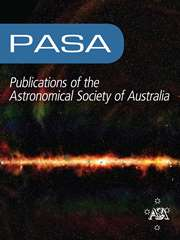Publications of the Astronomical Society of Australia

# The First Large Absorption Survey in H i (FLASH): I. Science goals and survey design

Published online by Cambridge University Press:  21 March 2022

*
Corresponding author: James R. Allison, email: james.allison@physics.ox.ac.uk

## Abstract

We describe the scientific goals and survey design of the First Large Absorption Survey in H i (FLASH), a wide field survey for 21-cm line absorption in neutral atomic hydrogen (H i) at intermediate cosmological redshifts. FLASH will be carried out with the Australian Square Kilometre Array Pathfinder (ASKAP) radio telescope and is planned to cover the sky south of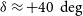$\delta \approx +40\,\deg$ at frequencies between 711.5 and 999.5 MHz. At redshifts between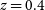$z = 0.4$ and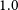$1.0$ (look-back times of 4 – 8 Gyr), the H i content of the Universe has been poorly explored due to the difficulty of carrying out radio surveys for faint 21-cm line emission and, at ultra-violet wavelengths, space-borne searches for Damped Lyman-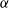$\alpha$ absorption in quasar spectra. The ASKAP wide field of view and large spectral bandwidth, in combination with a radio-quiet site, will enable a search for absorption lines in the radio spectra of bright continuum sources over 80% of the sky. This survey is expected to detect at least several hundred intervening 21-cm absorbers and will produce an H i-absorption-selected catalogue of galaxies rich in cool, star-forming gas, some of which may be concealed from optical surveys. Likewise, at least several hundred associated 21-cm absorbers are expected to be detected within the host galaxies of radio sources at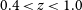$0.4 < z < 1.0$ , providing valuable kinematical information for models of gas accretion and jet-driven feedback in radio-loud active galactic nuclei. FLASH will also detect OH 18-cm absorbers in diffuse molecular gas, megamaser OH emission, radio recombination lines, and stacked H i emission.

## Keywords

Type
Research Article
© The Author(s), 2022. Published by Cambridge University Press on behalf of the Astronomical Society of Australia

## Access options

Get access to the full version of this content by using one of the access options below. (Log in options will check for institutional or personal access. Content may require purchase if you do not have access.)

## Footnotes

*

Jansky Fellow of the National Radio Astronomy Observatory

## References

Aalto, S., Booth, R. S., Black, J. H., & Johansson, L. E. B. 1995, A&A, 300, 369 Google Scholar
Adams, E. A. K., & van Leeuwen, J. 2019, NatAs, 3, 188 CrossRefGoogle Scholar
Aditya, J. N. H. S., & Kanekar, N. 2018a, MNRAS, 473, 59 CrossRefGoogle Scholar
Aditya, J. N. H. S., & Kanekar, N. 2018b, MNRAS, 481, 1578 CrossRefGoogle Scholar
Aditya, J. N. H. S., Kanekar, N., & Kurapati, S. 2016, MNRAS, 455, 4000 CrossRefGoogle Scholar
Aditya, J. N. H. S., Jorgenson, R., Joshi, V., Singh, V., An, T., & Chandola, Y. 2021, MNRAS, 500, 998 CrossRefGoogle Scholar
Agudo, I., et al. 2015, in Advancing Astrophysics with the Square Kilometre Array (AASKA14), 93 (arXiv:1501.00420)Google Scholar
Allen, S. W., Dunn, R. J. H., Fabian, A. C., Taylor, G. B., & Reynolds, C. S. 2006, MNRAS, 372, 21 Google Scholar
Allison, J. R. 2021, MNRAS, 503, 985 CrossRefGoogle Scholar
Allison, J. R., Sadler, E. M., & Whiting, M. T. 2012, PASA, 29, 221 CrossRefGoogle Scholar
Allison, J. R., Sadler, E. M., Meekin, A. M. 2014, MNRAS, 440, 696 CrossRefGoogle Scholar
Allison, J. R., et al. 2015, MNRAS, 453, 1249 Google Scholar
Allison, J. R., et al. 2016a, AN, 337, 175 Google Scholar
Allison, J. R., Zwaan, M. A., Duchesne, S. W., & Curran, S. J. 2016b, MNRAS, 462, 1341 CrossRefGoogle Scholar
Allison, J. R., et al. 2017, MNRAS, 465, 4450 Google Scholar
Allison, J. R., et al. 2019, MNRAS, 482, 2934 Google Scholar
Allison, J. R., et al. 2020, MNRAS, 494, 3627 Google Scholar
Alves, M. I., Calabretta, M., Davies, R. D., Dickinson, C., Staveley-Smith, L., Davis, R. J., Chen, T., & Barr, A. 2015, MNRAS, 450, 2025 CrossRefGoogle Scholar
An, T., & Baan, W. A. 2012, ApJ, 760, 77 CrossRefGoogle Scholar
Anantharamaiah, K. R. 1986, JApA, 7, 131 CrossRefGoogle Scholar
Anderson, C. S., Gaensler, B. M., Feain, I. J., & Franzen, T. M. O. 2015, ApJ, 815, 49 CrossRefGoogle Scholar
Anderson, L. D., et al. 2021, ApJSS, 254, 28 Google Scholar
Arshakian, T. G., Beck, R., Krause, M., & Sokoloff, D. 2009, A&A, 494, 21 CrossRefGoogle Scholar
Astropy Collaboration et al. 2013, A&A, 558, A33 CrossRefGoogle Scholar
Astropy Collaboration et al. 2018, AJ, 156, 123 Google Scholar
Baan, W. A., Rhoads, J., Fisher, K., Altschuler, D. R., & Haschick, A. 1992, ApJ, 396, L99 CrossRefGoogle Scholar
Bahcall, J. N., & Ekers, R. D. 1969, ApJ, 157, 1055 CrossRefGoogle Scholar
Bahé, Y. M., et al. 2016, MNRAS, 456, 1115 CrossRefGoogle Scholar
Balashev, S. A., & Noterdaeme, P. 2018, MNRAS, 478, L7 CrossRefGoogle Scholar
Balashev, S. A., Klimenko, V. V., Ivanchik, A. V., Varshalovich, D. A., Petitjean, P., & Noterdaeme, P. 2014, MNRAS, 440, 225 CrossRefGoogle Scholar
Balashev, S. A., et al. 2019, MNRAS, 490, 2668 CrossRefGoogle Scholar
Basu, A., Mao, S. A., Fletcher, A., Kanekar, N., Shukurov, A., Schnitzeler, D., Vacca, V., & Junklewitz, H. 2018, MNRAS, 477, 2528 CrossRefGoogle Scholar
Beck, R., 2009, Ap&SST, 5, 43 Google Scholar
Becker, R. H., White, R. L., & Helfand, D. J. 1995, ApJ, 450, 559 CrossRefGoogle Scholar
Bell, M. B., & Seaquist, E. R. 1980, ApJ, 238, 818 CrossRefGoogle Scholar
Bell, M. B., Seaquist, E. R., Mebold, U., Reif, K., & Shaver, P. A. 1984, A&A, 130, 1 Google Scholar
Bera, A., Kanekar, N., Chengalur, J. N., & Bagla, J. S. 2019, ApJ, 882, L7 CrossRefGoogle Scholar
Berg, T. A. M., et al. 2019, MNRAS, 488, 4356 CrossRefGoogle Scholar
Berger, M. J., & Colella, P. 1989, J. Comput. Phys., 82, 64 CrossRefGoogle Scholar
Berger, M. J., Oliger, J. 1984, J. Comput. Phys., 53, 484 CrossRefGoogle Scholar
Bernet, M. L., Miniati, F., Lilly, S. J., Kronberg, P. P., & Dessauges-Zavadsky, M. 2008, Nature, 454, 302 CrossRefGoogle Scholar
Berta, S., et al. 2013, A&A, 555, L8 CrossRefGoogle Scholar
Beuchert, T., et al. 2018, A&A, 612, L4 CrossRefGoogle Scholar
Bird, S., Garnett, R., & Ho, S. 2017, MNRAS, 466, 2111 CrossRefGoogle Scholar
Bland-Hawthorn, J., Maloney, P. R., Stephens, A., Zovaro, A., & Popping, A. 2017, ApJ, 849, 51 CrossRefGoogle Scholar
Blanton, M. R., et al. 2001, AJ, 121, 2358 Google Scholar
Blanton, M. R., et al. 2017, AJ, 154, 28 Google Scholar
Blyth, S., et al. 2016, in MeerKAT Science: On the Pathway to the SKA. Article ID: 4Google Scholar
Borthakur, S. 2016, ApJ, 829, 128 CrossRefGoogle Scholar
Borthakur, S., Tripp, T. M., Yun, M. S., Bowen, D. V., Meiring, J. D., York, D. G., & Momjian, E. 2011, ApJ, 727, 52 CrossRefGoogle Scholar
Braun, R. 2012, ApJ, 749, 87 CrossRefGoogle Scholar
Brentjens, M. A., & de Bruyn, A. G. 2005, A&A, 441, 1217 CrossRefGoogle Scholar
Brookes, M. H., Best, P. N., Peacock, J. A., Röttgering, H. J. A., & Dunlop, J. S. 2008, MNRAS, 385, 1297 CrossRefGoogle Scholar
Buchner, J., et al. 2014, A&A, 564, A125 CrossRefGoogle Scholar
Cannon, R., et al. 2006, MNRAS, 372, 425 Google Scholar
Carilli, C. L., Menten, K. M., Reid, M. J., Rupen, M. P., & Yun, M. S. 1998, ApJ, 494, 175 CrossRefGoogle Scholar
Catinella, B., & Cortese, L. 2015, MNRAS, 446, 3526 CrossRefGoogle Scholar
Cattaneo, A., et al. 2009, Nature, 460, 213 CrossRefGoogle Scholar
Chandola, Y., & Saikia, D. J. 2017, MNRAS, 465, 997 CrossRefGoogle Scholar
Chandola, Y., Sirothia, S. K., & Saikia, D. J. 2011, MNRAS, 418, 1787 CrossRefGoogle Scholar
Chandola, Y., Gupta, N., & Saikia, D. J. 2013, MNRAS, 429, 2380 CrossRefGoogle Scholar
Chandola, Y., Saikia, D. J., & Li, D. 2020, MNRAS, 494, 5161 CrossRefGoogle Scholar
Chang, T.-C., Pen, U.-L., Bandura, K., & Peterson, J. B. 2010, Nature, 466, 463 CrossRefGoogle Scholar
Chengalur, J. N., & Kanekar, N. 2003, Phys. Rev. Lett., 91, 241302 CrossRefGoogle Scholar
Chengalur, J. N., de Bruyn, A. G., & Narasimha, D. 1999, A&A, 343, L79 Google Scholar
Ching, J. H. Y., et al. 2017, MNRAS, 464, 1306 CrossRefGoogle Scholar
Chippendale, A. P., et al. 2015, in 2015 International Conference on Electromagnetics in Advanced Applications (ICEAA). IEEE, 541–544 (arXiv:1509.00544)Google Scholar
Chowdhury, A., Kanekar, N., Chengalur, J. N., Sethi, S., & Dwarakanath, K. S. 2020a, Nature, 586, 369 CrossRefGoogle Scholar
Chowdhury, A., Kanekar, N., & Chengalur, J. N. 2020b, ApJ, 900, L30 CrossRefGoogle Scholar
Chowdhury, A., Kanekar, N., Das, B., Dwarakanath, K. S., & Sethi, S. 2021, ApJ, 913, L24 CrossRefGoogle Scholar
Churchwell, E., & Shaver, P. A. 1979, A&A, 77, 316 Google Scholar
Condon, J. J. 1984, ApJ, 287, 461 CrossRefGoogle Scholar
Condon, J. J. 1992, ARAA, 30, 575 CrossRefGoogle Scholar
Condon, J. J., Cotton, W. D., Greisen, E. W., Yin, Q. F., Perley, R. A., Taylor, G. B., & Broderick, J. J. 1998, AJ, 115, 1693 CrossRefGoogle Scholar
Cornwell, T., Humphreys, B., Lenc, E., Voronkov, M., & Whiting, M. 2011, ATNF ASKAP Memorandum 27, 167 Google Scholar
Crain, R. A., et al. 2017, MNRAS, 464, 4204 CrossRefGoogle Scholar
Crighton, N. H. M., et al. 2015, MNRAS, 452, 217 CrossRefGoogle Scholar
Croton, D. J., et al. 2006, MNRAS, 365, 11 CrossRefGoogle Scholar
Curran, S. J. 2019, MNRAS, 484, 3911 CrossRefGoogle Scholar
Curran, S. J. 2020, A&A, 635, A166 CrossRefGoogle Scholar
Curran, S. J. 2021, MNRAS, 506, 1548 CrossRefGoogle Scholar
Curran, S. J., & Whiting, M. T. 2010, ApJ, 712, 303 CrossRefGoogle Scholar
Curran, S. J., & Whiting, M. T. 2012, ApJ, 759, 117 CrossRefGoogle Scholar
Curran, S. J., Kanekar, N., & Darling, J. K. 2004a, New Astro. Reviews, 48, 1095 CrossRefGoogle Scholar
Curran, S. J., Murphy, M. T., Pihlström, Y. M., Webb, J. K., Bolatto, A. D., & Bower, G. C. 2004b, MNRAS, 352, 563 CrossRefGoogle Scholar
Curran, S. J., Whiting, M. T., Murphy, M. T., Webb, J. K., Longmore, S. N., Pihlström, Y. M., Athreya, R., & Blake, C. 2006, MNRAS, 371, 431 CrossRefGoogle Scholar
Curran, S. J., Darling, J., Bolatto, A. D., Whiting, M. T., Bignell, C., & Webb, J. K. 2007, MNRAS, 382, L11 CrossRefGoogle Scholar
Curran, S. J., Whiting, M. T., Wiklind, T., Webb, J. K., Murphy, M. T., & Purcell, C. R. 2008, MNRAS, 391, 765 CrossRefGoogle Scholar
Curran, S. J., et al. 2011a, MNRAS, 413, 1165 Google Scholar
Curran, S. J., et al. 2011b, MNRAS, 416, 2143 CrossRefGoogle Scholar
Curran, S. J., Whiting, M. T., Sadler, E. M., & Bignell, C. 2013, MNRAS, 428, 2053 CrossRefGoogle Scholar
Curran, S. J., Allison, J. R., Whiting, M. T., Sadler, E. M., Combes, F., Pracy, M. B., Bignell, C., & Athreya, R. 2016a, MNRAS, 457, 3666 CrossRefGoogle Scholar
Curran, S. J., Reeves, S. N., Allison, J. R., & Sadler, E. M. 2016b, MNRAS, 459, 4136 CrossRefGoogle Scholar
Curran, S. J., Duchesne, S. W., Divoli, A., & Allison, J. R. 2016c, MNRAS, 462, 4197 CrossRefGoogle Scholar
Curran, S. J., Whiting, M. T., Allison, J. R., Tanna, A., Sadler, E. M., & Athreya, R. 2017, MNRAS, 467, 4514 CrossRefGoogle Scholar
Curran, S. J., Hunstead, R. W., Johnston, H. M., Whiting, M. T., Sadler, E. M., Allison, J. R., & Athreya, R. 2019, MNRAS, 484, 1182 CrossRefGoogle Scholar
Cutri, R. M., et al. 2021, VizieR Online Data Catalog, p. II/328 Google Scholar
Collaboration, DESI, et al. 2016 (arXiv:1611.00036)Google Scholar
Darling, J. 2003, Phys. Rev. Lett., 91, 011301 CrossRefGoogle Scholar
Darling, J. 2007, ApJL, 669, L9 CrossRefGoogle Scholar
Darling, J., & Giovanelli, R., 2002, ApJ, 572, 810 CrossRefGoogle Scholar
Darling, J., Giovanelli, R., Haynes, M. P., Bolatto, A. D., & Bower, G. C., 2004, ApJ, 613, L101 CrossRefGoogle Scholar
Darling, J., Macdonald, E. P., Haynes, M. P., & Giovanelli, R. 2011, ApJ, 742, 60 CrossRefGoogle Scholar
Davé, R., Crain, R. A., Stevens, A. R. H., Narayanan, D., Saintonge, A., Catinella, B., & Cortese, L. 2020, MNRAS, 497, 146 CrossRefGoogle Scholar
De Zotti, G., Massardi, M., Negrello, M., & Wall, J. 2010, A&AR, 18, 1 CrossRefGoogle Scholar
DeBoer, D. R., et al. 2009, IEEE Proc., 97, 1507 CrossRefGoogle Scholar
Decarli, R., et al. 2019, ApJ, 882, 138 Google Scholar
Decarli, R., et al., 2020, ApJ, 902, 110 CrossRefGoogle Scholar
Delhaize, J., Meyer, M. J., Staveley-Smith, L., & Boyle, B. J. 2013, MNRAS, 433, 1398 CrossRefGoogle Scholar
Di Matteo, T., Springel, V., & Hernquist, L. 2005, Nature, 433, 604 CrossRefGoogle Scholar
Dickey, J. M., Strasser, S., Gaensler, B. M., Haverkorn, M., Kavars, D., McClure-Griffiths, N. M., Stil, J., & Taylor, A. R. 2009, ApJ, 693, 1250 CrossRefGoogle Scholar
Diemer, B., et al. 2019, MNRAS, 487, 1529 CrossRefGoogle Scholar
Drinkwater, M. J., Webb, J. K., Barrow, J. D., & Flambaum, V. V. 1998, MNRAS, 295, 457 CrossRefGoogle Scholar
Drinkwater, M. J., et al. 2010, MNRAS, 401, 1429 Google Scholar
Driver, S. P., et al. 2018, MNRAS, 475, 2891 Google Scholar
Dutta, R., Srianand, R., Gupta, N., Momjian, E., Noterdaeme, P., Petitjean, P., & Rahmani, H. 2017a, MNRAS, 465, 588 CrossRefGoogle Scholar
Dutta, R., Srianand, R., Gupta, N., Joshi, R., Petitjean, P., Noterdaeme, P., Ge, J., & Krogager, J. K. 2017b, MNRAS, 465, 4249 CrossRefGoogle Scholar
Dutta, R., Srianand, R., & Gupta, N. 2018, MNRAS, 480, 947 CrossRefGoogle Scholar
Dutta, R., Srianand, R., & Gupta, N. 2019, MNRAS, 489, 1099 CrossRefGoogle Scholar
Dutta, R., Raghunathan, S., Gupta, N., & Joshi, R. 2020, MNRAS, 491, 838 CrossRefGoogle Scholar
Emig, K. L., Salas, P., de Gasperin, F., Oonk, J. B. R., Toribio, M. C., Rottgering, H. J. A., & Tielens, A. G. G. M. 2019, A&A, 622, A7 CrossRefGoogle Scholar
Emig, K. L., Salas, P., de Gasperin, F., Oonk, J. B. R., Toribio, M. C., Mechev, A. P., Rottgering, H. J. A., & Tielens, A. G. G. M. 2020, A&A, 634, 138 CrossRefGoogle Scholar
Fabian, A. C. 2012, ARA&A, 50, 455 CrossRefGoogle Scholar
Farnes, J. S., O’Sullivan, S. P., Corrigan, M. E., & Gaensler, B. M. 2014, ApJ, 795, 63 CrossRefGoogle Scholar
Fernández, X., et al. 2016, ApJ, 824, L1 Google Scholar
Feroz, F., & Hobson, M. P., 2008, MNRAS, 384, 449 CrossRefGoogle Scholar
Feroz, F., Hobson, M. P., & Bridges, M. 2009, MNRAS, 398, 1601 CrossRefGoogle Scholar
Feroz, F., Hobson, M. P., Cameron, E., & Pettitt, A. N. 2013 (arXiv:1306.2144)Google Scholar
Ferrarese, L., & Merritt, D. 2000, ApJ, 539, L9CrossRefGoogle Scholar
Ferrière, K., & Schmitt, D. 2000, A&A, 358, 125 Google Scholar
Ferriére, K., West, J. L., & Jaffe, T. R. 2021, MNRAS,Google Scholar
Field, G. B. 1958, Proc. IRE, 46, 240 CrossRefGoogle Scholar
Field, G. B. 1959, ApJ, 129, 536 CrossRefGoogle Scholar
Fletcher, T. J., Saintonge, A., Soares, P. S., & Pontzen, A. 2021, MNRAS, 501, 411 CrossRefGoogle Scholar
Furlanetto, S. R., & Loeb, A. 2001, ApJ, 556, 619 CrossRefGoogle Scholar
Gabuzda, D. 2018, Galaxies, 7, 5 CrossRefGoogle Scholar
Gaensler, B. M., Landecker, T. L., Taylor, A. R., & POSSUM Collaboration, 2010, in American Astronomical Society Meeting Abstracts #215. p. 470.13Google Scholar
Garnett, R., Ho, S., Bird, S., & Schneider, J. 2017, MNRAS, 472, 1850 CrossRefGoogle Scholar
Garratt-Smithson, L., Power, C., Lagos, C. d. P., Stevens, A. R. H., Allison, J. R., & Sadler, E. M. 2021, MNRAS, 501, 4396 CrossRefGoogle Scholar
Genzel, R., et al. 2015, ApJ, 800, 20 Google Scholar
Geréb, K., Maccagni, F. M., Morganti, R., & Oosterloo, T. A. 2015, A&A, 575, A44 CrossRefGoogle Scholar
Gingold, R. A., & Monaghan, J. J. 1977, MNRAS, 181, 375 CrossRefGoogle Scholar
Giovanelli, R., et al. 2005, AJ, 130, 2598 CrossRefGoogle Scholar
Glowacki, M., et al. 2017, MNRAS, 467, 2766 Google Scholar
Glowacki, M., et al. 2019, MNRAS, 489, 4926 CrossRefGoogle Scholar
Goldsmith, P. F., Yildiz, U. A., Langer, W. D., & Pineda, J. L. 2015, ApJ, 814, 133 CrossRefGoogle Scholar
Grasha, K., Darling, J., Bolatto, A., Leroy, A. K., & Stocke, J. T. 2019, ApJS, 245, 3 CrossRefGoogle Scholar
Grasha, K., Darling, J., Leroy, A. K., & Bolatto, A. D. 2020, MNRAS, 498, 883 CrossRefGoogle Scholar
Guélin, M., & Weliachew, L. 1970, A&A, 9, 155 Google Scholar
Gupta, N., Salter, C. J., Saikia, D. J., Ghosh, T., & Jeyakumar, S. 2006, MNRAS, 373, 972 CrossRefGoogle Scholar
Gupta, N., Srianand, R., Petitjean, P., Noterdaeme, P., & Saikia, D. J. 2009, MNRAS, 398, 201 CrossRefGoogle Scholar
Gupta, N., Srianand, R., Bowen, D. V., York, D. G., & Wadadekar, Y. 2010, MNRAS, 408, 849 CrossRefGoogle Scholar
Gupta, N., Srianand, R., Petitjean, P., Bergeron, J., Noterdaeme, P., & Muzahid, S. 2012, A&A, 544, A21 CrossRefGoogle Scholar
Gupta, N., et al., 2016, in MeerKAT Science: On the Pathway to the SKA, 14 (arXiv:1708.07371)Google Scholar
Gupta, N., Momjian, E., Srianand, R., Petitjean, P., Noterdaeme, P., Gyanchandani, D., Sharma, R., & Kulkarni, S. 2018, ApJ, 860, L22 CrossRefGoogle Scholar
Gupta, N., et al. 2021, ApJ, 907, 11 Google Scholar
Hanasz, M., Wóltański, D., & Kowalik, K. 2009, ApJ, 706, L155 CrossRefGoogle Scholar
Hay, S. G., & O’Sullivan, J. D. 2008, Radio Sci., 43, 6 CrossRefGoogle Scholar
Heckman, T. M., & Best, P. N. 2014, ARA&A, 52, 589 Google Scholar
Heckman, T. M., Smith, E. P., Baum, S. A., van Breugel, W. J. M., Miley, G. K., Illingworth, G. D., Bothun, G. D., & Balick, B. 1986, ApJ, 311, 526 CrossRefGoogle Scholar
Heiles, C., & Troland, T. H. 2003, ApJ, 586, 1067 CrossRefGoogle Scholar
Heintz, K. E., Watson, D., Oesch, P. A., Narayanan, D., & Madden, S. C. 2021, ApJ, 922, 147 CrossRefGoogle Scholar
Hellwig, H., Vessot, R., Levine, M., Zitzewitz, W., P., W. Allan, D., & J. Glaze, D. 1970, IEEE Trans. Instrument. Measur., 19, 200 CrossRefGoogle Scholar
Henkel, C., Jethava, N., Kraus, A., Menten, K. M., Carilli, C. L., Grasshoff, M., Lubowich, D., & Reid, M. J. 2005, A&A, 440, 893 CrossRefGoogle Scholar
Hickox, R. C., & Alexander, D. M. 2018, ARA&A, 56, 625 CrossRefGoogle Scholar
Hopkins, A. M., & Beacom, J. F. 2006, ApJ, 651, 142 CrossRefGoogle Scholar
Hoppmann, L., Staveley-Smith, L., Freudling, W., Zwaan, M. A., Minchin, R. F., & Calabretta, M. R. 2015, MNRAS, 452, 3726 CrossRefGoogle Scholar
Hotan, A. W., et al. 2021, PASA, 38, E009 Google Scholar
Ideguchi, S., Miyashita, Y., & Heald, G. 2018, Galaxies, 6 CrossRefGoogle Scholar
Israel, F. P. 1988, in C and$\textrm{H}_{2}$ in Galaxies, ed. R. D. Wolstencroft & W. B. Burton, vol. 147, p. 281. 10.1007/978-94-009-3019-3_10 CrossRefGoogle Scholar
Ivezić, Ž., et al. 2019, ApJ, 873, 111 Google Scholar
Ivison, R. J., et al. 2012, MNRAS, 425, 1320 Google Scholar
Johnston, S., et al. 2008, ExA, 22, 151 CrossRefGoogle Scholar
Jonas, J., & MeerKAT Team 2016, in MeerKAT Science: On the Pathway to the SKA, 1 Google Scholar
Jones, M. G., Haynes, M. P., Giovanelli, R., & Moorman, C. 2018, MNRAS, 477, 2 CrossRefGoogle Scholar
Kanekar, N., & Chengalur, J. N. 2002, A&A, 381, L73 CrossRefGoogle Scholar
Kanekar, N., Chengalur, J. N., de Bruyn, A. G., & Narasimha, D. 2003, MNRAS, 345, L7 CrossRefGoogle Scholar
Kanekar, N., et al. 2005, Phys. Rev. Lett., 95, 261301 Google Scholar
Kanekar, N., Prochaska, J. X., Ellison, S. L., & Chengalur, J. N. 2009, MNRAS, 396, 385 CrossRefGoogle Scholar
Kanekar, N., et al. 2014a, MNRAS, 438, 2131 CrossRefGoogle Scholar
Kanekar, N., Gupta, A., Carilli, C. L., Stocke, J. T., & Willett, K. W. 2014b, ApJ, 782, 56 CrossRefGoogle Scholar
Kass, R. E., & Raftery, A. E. 1995, J. Amer. Stat. Assoc., 90, 773 CrossRefGoogle Scholar
Kauffmann, G., et al. 2003, MNRAS, 346, 1055 CrossRefGoogle Scholar
Kim, K. S., Lilly, S. J., Miniati, F., Bernet, M. L., Beck, R., O’Sullivan, S. P., & Gaensler, B. M. 2016, ApJ, 829, 133 CrossRefGoogle Scholar
Klamer, I. J., Ekers, R. D., Sadler, E. M., & Hunstead, R. W. 2004, ApJ, 612, L97 CrossRefGoogle Scholar
Koribalski, B. S., et al. 2020, Ap&SS, 365, 118 Google Scholar
Kormendy, J., & Ho, L. C. 2013, ARA&A, 51, 511 CrossRefGoogle Scholar
Kormendy, J., & Richstone, D. 1995, ARA&A, 33, 581 CrossRefGoogle Scholar
Kronberg, P. P., Lesch, H., & Hopp, U. 1999, ApJ, 511, 56 CrossRefGoogle Scholar
Krumholz, M. R., McKee, C. F., & Tumlinson, J. 2008, ApJ, 689, 865 CrossRefGoogle Scholar
Krumholz, M. R., McKee, C. F., & Tumlinson, J. 2009a, ApJ, 693, 216 CrossRefGoogle Scholar
Krumholz, M. R., McKee, C. F., & Tumlinson, J. 2009b, ApJ, 699, 850 CrossRefGoogle Scholar
Lah, P., et al. 2007, MNRAS, 376, 1357 Google Scholar
Lah, P., et al. 2009, MNRAS, 399, 1447 CrossRefGoogle Scholar
Lane, W. M. 2000, PhD thesis, University of GroningenGoogle Scholar
Lane, W. M., & Briggs, F. H. 2001, ApJ, 561, L27 CrossRefGoogle Scholar
Lenkić, L., et al. 2020, AJ, 159, 190 CrossRefGoogle Scholar
Levshakov, S. A., & Varshalovich, D. A. 1985, MNRAS, 212, 517 CrossRefGoogle Scholar
Li, L.-C., Staveley-Smith, L., & Rhee, J. 2021, RAA, 21, 030 CrossRefGoogle Scholar
Liske, J., et al. 2015, MNRAS, 452, 2087 Google Scholar
Liszt, H. 2001, A&A, 371, 698 CrossRefGoogle Scholar
Lucy, L. B. 1977, AJ, 82, 1013 CrossRefGoogle Scholar
Maccagni, F. M., Morganti, R., Oosterloo, T. A., & Mahony, E. K. 2014, A&A, 67, 4 CrossRefGoogle Scholar
Maccagni, F. M., Morganti, R., Oosterloo, T. A., Geréb, K., & Maddox, N. 2017, A&A, 604, A43 CrossRefGoogle Scholar
Macquart, J.-P., & Tingay, S. 2016, MNRAS, 460, 2322 CrossRefGoogle Scholar
Maddox, N., Hess, K. M., Obreschkow, D., Jarvis, M. J., & Blyth, S. L. 2015, MNRAS, 447, 1610 CrossRefGoogle Scholar
Magnelli, B., et al. 2020, ApJ, 892, 66 Google Scholar
Magorrian, J., et al. 1998, AJ, 115, 2285 CrossRefGoogle Scholar
Mahony, E. K., Morganti, R., Emonts, B. H. C., Oosterloo, T. A., & Tadhunter, C. 2013, MNRAS Letters, 435, 58 CrossRefGoogle Scholar
Mahony, E. K., Oonk, J. B. R., Morganti, R., Tadhunter, C., Bessiere, P., Short, P., Emonts, B. H. C., & Oosterloo, T. A. 2016, MNRAS, 2460, 2453 CrossRefGoogle Scholar
Mahony, E. K., et al. 2022, MNRAS, 509, 1690 Google Scholar
Mao, S. A., et al. 2017, Nature Astronomy, 1, 621 CrossRefGoogle Scholar
Masui, K. W., et al. 2013, ApJ, 763, L20 CrossRefGoogle Scholar
McBride, J., & Heiles, C. 2013, ApJ, 763, 8 CrossRefGoogle Scholar
McConnell, D., et al. 2016, PASA, 33, e042 Google Scholar
McConnell, D., et al. 2020, PASA, 37, e048 CrossRefGoogle Scholar
McKee, C. F., & Krumholz, M. R. 2010, ApJ, 709, 308 CrossRefGoogle Scholar
McKee, C. F., & Ostriker, E. C. 2007, AR&A, 45, 565 CrossRefGoogle Scholar
Merloni, A., et al. 2012 (arXiv:1209.3114)Google Scholar
Mhaskey, M., Paul, S., Gupta, N., Mukherjee, D., & Gopal-Krishna, 2020, A&A, 643, A174 CrossRefGoogle Scholar
Molaro, P., Bonifacio, P., Centurión, M., D’Odorico, S., Vladilo, G., Santin, P., & Di Marcantonio, P. 2000, ApJ, 541, 54 CrossRefGoogle Scholar
Morganti, R., & Oosterloo, T. 2018, A&ARv, 26, 1 CrossRefGoogle Scholar
Morganti, R., Oosterloo, T., & Tsvetanov, Z. 1998, AJ, 115, 915 CrossRefGoogle Scholar
Morganti, R., Oosterloo, T. A., Tadhunter, C. N., van Moorsel, G., Killeen, N., & Wills, K. A. 2001, MNRAS, 323, 331 CrossRefGoogle Scholar
Morganti, R., Tadhunter, C. N., & Oosterloo, T. A. 2005, A&A, 444, L9 CrossRefGoogle Scholar
Morganti, R., Fogasy, J., Paragi, Z., Oosterloo, T., & Orienti, M. 2013, Science, 341, 1082 CrossRefGoogle Scholar
Moss, V. A., et al. 2017, MNRAS, 471, 2952 Google Scholar
Muller, S., et al. 2013, A&A, 551, A109 CrossRefGoogle Scholar
Murray, C. E., Stanimirović, S., Goss, W. M., Heiles, C., Dickey, J. M., Babler, B., & Kim, C.-G. 2018, ApJS, 238, 14 CrossRefGoogle Scholar
Murthy, S., Morganti, R., Oosterloo, T., & Maccagni, F. M. 2021, A&A, 654, A94 CrossRefGoogle Scholar
Neeleman, M., Prochaska, J. X., Ribaudo, J., Lehner, N., Howk, J. C., Rafelski, M., & Kanekar, N. 2016, ApJ, 818, 113 CrossRefGoogle Scholar
Nelson, D., et al. 2020, MNRAS, 498, 2391 CrossRefGoogle Scholar
Nesvadba, N. P. H., et al. 2009, MNRAS, 395, L16 CrossRefGoogle Scholar
Norris, R. P., et al. 2011, PASA, 28, 215 CrossRefGoogle Scholar
Norris, R. P., et al. 2021, PASA, 38, E046 Google Scholar
Noterdaeme, P., et al. 2012, A&A, 547, L1 Google Scholar
Noterdaeme, P., Srianand, R., Rahmani, H., Petitjean, P., Pâris, I., Ledoux, C., Gupta, N., & López, S. 2015, A&A, 577, A24 CrossRefGoogle Scholar
Noterdaeme, P., et al. 2017, A&A, 597, A82 CrossRefGoogle Scholar
O’Dea, C. P., & Saikia, D. J. 2021, AR&Av, 29, 3 Google Scholar
O’Sullivan, S. P., et al. 2012, MNRAS, 421, 3300 CrossRefGoogle Scholar
O’Sullivan, S. P., Gaensler, B. M., Lara-López, M. A., van Velzen, S., Banfield, J. K., & Farnes, J. S. 2015, ApJ, 806, 83 CrossRefGoogle Scholar
O’Sullivan, S. P., Purcell, C. R., Anderson, C. S., Farnes, J. S., Sun, X. H., & Gaensler, B. M. 2017, MNRAS, 469, 4034 CrossRefGoogle Scholar
Omont, A., Petitjean, P., Guilloteau, S., McMahon, R. G., Solomon, P. M., & Pécontal, E. 1996, Nature, 382, 428 CrossRefGoogle Scholar
Oonk, J. B. R., van Weeren, R. J., Salas, P., Salgado, F., Morabito, L. K., Toribio, M. C., Tielens, A. G. G. M., & Röttgering, H. J. A. 2017, MNRAS, 465, 1066 CrossRefGoogle Scholar
Oren, A. L., & Wolfe, A. M. 1995, ApJ, 445, 624 CrossRefGoogle Scholar
Orienti, M., Morganti, R., & Dallacasa, D. 2006, A&A, 457, 531 CrossRefGoogle Scholar
Ostorero, L., et al. 2010, ApJ, 715, 1071 CrossRefGoogle Scholar
Ostorero, L., Morganti, R., Diaferio, A., Siemiginowska, A., Stawarz, Ł., Moderski, R., & Labiano, A. 2017, ApJ, 849, 34 CrossRefGoogle Scholar
Papadopoulos, P. P., Röttgering, H. J. A., van der Werf, P. P., Guilloteau, S., Omont, A., van Breugel, W. J. M., & Tilanus, R. P. J. 2000, ApJ, 528, 626 CrossRefGoogle Scholar
Péroux, C., & Howk, J. C. 2020, ARA&A, 58, 363 CrossRefGoogle Scholar
Pihlström, Y. M., Conway, J. E., & Vermeulen, R. C. 2003, A&A, 404, 871 CrossRefGoogle Scholar
Pracy, M. B., et al. 2016, MNRAS, 17, 2 Google Scholar
Purcell, E. M., & Field, G. B. 1956, ApJ, 124, 542 CrossRefGoogle Scholar
Ranjan, A., Noterdaeme, P., Krogager, J. K., Petitjean, P., Srianand, R., Balashev, S. A., Gupta, N., & Ledoux, C. 2020, A&A, 633, A125 CrossRefGoogle Scholar
Rao, S. M., Turnshek, D. A., Sardane, G. M., & Monier, E. M. 2017, MNRAS, 471, 3428 CrossRefGoogle Scholar
Reeves, S. N., Sadler, E. M., Allison, J. R., Koribalski, B. S., Curran, S. J., & Pracy, M. B. 2015, MNRAS, 450, 926 CrossRefGoogle Scholar
Reeves, S. N., et al. 2016, MNRAS, 457, 2613 CrossRefGoogle Scholar
Rhee, J., Zwaan, M. A., Briggs, F. H., Chengalur, J. N., Lah, P., Oosterloo, T., & van der Hulst, T. 2013, MNRAS, 435, 2693 CrossRefGoogle Scholar
Rhee, J., Lah, P., Chengalur, J. N., Briggs, F. H., & Colless, M. 2016, MNRAS, 460, 2675 CrossRefGoogle Scholar
Rhee, J., Lah, P., Briggs, F. H., Chengalur, J. N., Colless, M., Willner, S. P., Ashby, M. L. N., & Le Fèvre, O. 2018, MNRAS, 473, 1879 CrossRefGoogle Scholar
Riechers, D. A., et al. 2020a, ApJ, 895, 81 CrossRefGoogle Scholar
Riechers, D. A., et al. 2020b, ApJL, 896, L21 Google Scholar
Rieder, M., & Teyssier, R. 2016, MNRAS, 457, 1722 CrossRefGoogle Scholar
Roberts, M. S. 1970, ApJ, 161, L9 CrossRefGoogle Scholar
Roberts, H., Darling, J., & Baker, A. J. 2021, ApJ, 911, 38 CrossRefGoogle Scholar
Robishaw, T., Quataert, E., & Heiles, C. 2008, ApJ, 680, 981 CrossRefGoogle Scholar
Rodírguez-Puebla, A., Calette, A. R., Avila-Reese, V., Rodriguez-Gomez, V., & Huertas-Company, M. 2020, PASA, 37, e024 CrossRefGoogle Scholar
Saikia, D. J., & Gupta, N. 2003, A&A, 405, 499 CrossRefGoogle Scholar
Salas, P., et al. 2017, MNRAS, 467, 2274 Google Scholar
Salgado, F., Morabito, L. K., Oonk, J. B. R., Salas, P., Toribio, M. C., Röttgering, H. J. A., & Tielens, A. G. G. M. 2017, ApJ, 837, 141 CrossRefGoogle Scholar
Sánchez-Ramírez, R., et al. 2016, MNRAS, 456, 4488 CrossRefGoogle Scholar
Schaye, J. 2001, ApJ, 562, L95 CrossRefGoogle Scholar
Schaye, J., et al. 2015, MNRAS, 446, 521 Google Scholar
Scoville, N., et al. 2017, ApJ, 837, 150 CrossRefGoogle Scholar
Shaver, P. A. 1975, Pramana, 5, 1 CrossRefGoogle Scholar
Shaver, P. A. 1978, A&A, 68, 97 CrossRefGoogle Scholar
Sokoloff, D. D., Bykov, A. A., Shukurov, A., Berkhuijsen, E. M., Beck, R., & Poezd, A. D. 1998, MNRAS, 299, 189 CrossRefGoogle Scholar
Somerville, R. S., & Davé, R. 2015, ARA&A, 53, 51 CrossRefGoogle Scholar
Springel, V. 2010, MNRAS, 401, 791 CrossRefGoogle Scholar
Srianand, R., Noterdaeme, P., Ledoux, C., & Petitjean, P. 2008, A&A, 482, L39 CrossRefGoogle Scholar
Tacconi, L. J., Genzel, R., & Sternberg, A. 2020, ARA&A, 58, 157 CrossRefGoogle Scholar
Tadhunter, C., Morganti, R., Rose, M., Oonk, J. B. R., & Oosterloo, T. 2014, Nature, 511, 440 CrossRefGoogle Scholar
Taylor, A. R., Stil, J. M., & Sunstrum, C. 2009, ApJ, 702, 1230 CrossRefGoogle Scholar
Teng, S. H., Veilleux, S., & Baker, A. J. 2013, ApJ, 765, 95 CrossRefGoogle Scholar
Tingay, S. J., et al. 2015, AJ, 149, 74 Google Scholar
Tremblay, G. R., et al. 2016, Nature, 534, 218Google Scholar
Tzanavaris, P., Webb, J. K., Murphy, M. T., Flambaum, V. V., & Curran, S. J. 2005, Phys. Rev. Lett., 95, 041301 CrossRefGoogle Scholar
Ursini, F., Bassani, L., Panessa, F., Bruni, G., Bazzano, A., Bird, A. J., Malizia, A., & Ubertini, P. 2019, Mem. Soc. Astron. Italiana, 90, 170 Google Scholar
Vermeulen, R. C., et al. 2003, A&A, 404, 861 Google Scholar
Veron-Cetty, M. P., Woltjer, L., Ekers, R. D., & Staveley-Smith, L. 1995, A&A, 297, L79 Google Scholar
Véron-Cetty, M. P., Woltjer, L., Staveley-Smith, L., & Ekers, R. D. 2000, A&A, 362, 426 Google Scholar
Vink, J., Snellen, I., Mack, K.-H., & Schilizzi, R. 2006, MNRAS, 367, 928 CrossRefGoogle Scholar
Walter, F., et al. 2020, ApJ, 902, 111 Google Scholar
Wang, J., Koribalski, B. S., Serra, P., van der Hulst, T., Roychowdhury, S., Kamphuis, P., & Chengalur, J. N. 2016, MNRAS, 460, 2143 CrossRefGoogle Scholar
Weinberger, R., Ehlert, K., Pfrommer, C., Pakmor, R., & Springel, V. 2017, MNRAS, 470, 4530 CrossRefGoogle Scholar
Whiting, M., & Humphreys, B. 2012, PASA, 29, 371 CrossRefGoogle Scholar
Wieringa, M., Raja, W., & Ord, S. 2020, in Pizzo R., Deul E. R., Mol J. D., de Plaa J., & Verkouter H., eds, Astronomical Society of the Pacific Conference Series Vol. 527, Astronomical Society of the Pacific Conference Series, 591 Google Scholar
Wiklind, T., & Combes, F. 1995, A&A, 299, 382 Google Scholar
Wiklind, T., & Combes, F. 1996a, A&A, 315, 86 CrossRefGoogle Scholar
Wiklind, T., & Combes, F. 1996b, Nature, 379, 139 CrossRefGoogle Scholar
Wiklind, T., & Combes, F. 1997, A&A, 328, 48 Google Scholar
Wiklind, T., & Combes, F. 2001, in Brainerd, T. G., & Kochanek, C. S., eds, Astronomical Society of the Pacific Conference Series Vol. 237, Gravitational Lensing: Recent Progress and Future Go. p. 155 (arXiv:astro-ph/9909314)Google Scholar
Wilman, R. J., et al. 2008, MNRAS, 388, 1335 Google Scholar
Wolfe, A. M., Jorgenson, R. A., Robishaw, T., Heiles, C., & Prochaska, J. X. 2011, ApJ, 733, 24 CrossRefGoogle Scholar
Wolfire, M. G., McKee, C. F., Hollenbach, D., & Tielens, A. G. G. M. 2003, ApJ, 587, 278 CrossRefGoogle Scholar
Wouthuysen, S. A. 1952, AJ, 57, 31 CrossRefGoogle Scholar
Yan, T., Stocke, J. T., Darling, J., Momjian, E., Sharma, S., & Kanekar, N. 2016, AJ, 151, 74 CrossRefGoogle Scholar
Zafar, T., Péroux, C., Popping, A., Milliard, B., Deharveng, J.-M., & Frank, S. 2013, A&A, 556, A141 CrossRefGoogle Scholar
Zhang, B., Zhu, M., Wu, Z.-Z., Yu, Q.-Z., Jiang, P., Yue, Y.-L., Huang, M.-L., & Hao, Q.-L. 2021, MNRAS, 503, 5385 CrossRefGoogle Scholar
Zwaan, M. A., & Prochaska, J. X. 2006, ApJ, 643, 675 CrossRefGoogle Scholar
Zwaan, M. A., Meyer, M. J., Staveley-Smith, L., & Webster, R. L. 2005a, MNRAS, 359, L30 CrossRefGoogle Scholar
Zwaan, M. A., Meyer, M. J., Staveley-Smith, L., & Webster, R. L. 2005b, MNRAS, 359, L30 CrossRefGoogle Scholar
Zwaan, M. A., van der Hulst, J. M., Briggs, F. H., Verheijen, M. A. W., & Ryan-Weber, E. V. 2005c, MNRAS, 364, 1467 CrossRefGoogle Scholar
van Cappellen, W. A., et al. 2021, in press, preprint (arXiv:2109.14234)Google Scholar